### Home > CAAC > Chapter 4 > Lesson 4.1.6 > Problem4-55

4-55.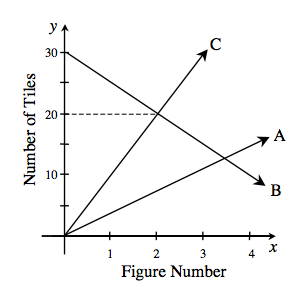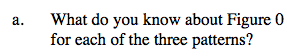What is the starting point for each pattern?

Figure 0 has
Pattern A: 0 tiles
Pattern B: 30 tiles
Pattern C: 0 tiles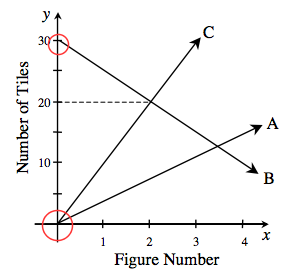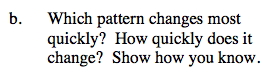Find the growth of each pattern.
For the pattern that changes the most quickly,
the growth will be the greatest.

Pattern C grows the most quickly at 10 tiles per figure.
Its line is the steepest.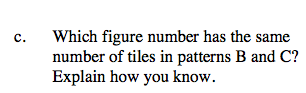Where two lines on the graph intersect, the number of tiles and the figure number are the same for those two patterns.

Figure 2 (See the graph).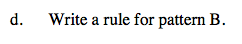Use the starting point for pattern B you found in part (a) and the growth you found for pattern B in part (b).